TREATING EACH TRANCHE AS A SEPARATE SECURITY

The preceding formulas can be used to price a multi-tranche MBS by treating each tranche as though it were a separate security. For example, the monthly payment on a tranche maturing five months before maturity, M, can be calculated using the formula: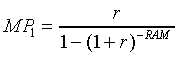Following this approach through all the cash flow equations allows us to calculate a price for the tranche.

Once we obtain prices for each tranche, we calculate the MBS price as an average of the individual tranche prices weighted by the dollar amount of the tranches: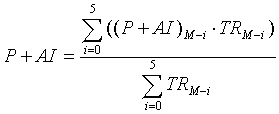where

TRM-i=remaining principal balance at k=0 of the trache maturing i months before maturity, M.

VAL01 is also calculated as an average of the tranche VAL01s weighted by the dollar amount of the tranches.

The risk measures Duration and Convexity are calculated as above. However, instead of weighting by outstanding face value TRM-i, we weight by market value: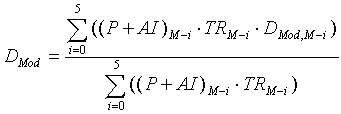Similarly, convexity is calculated: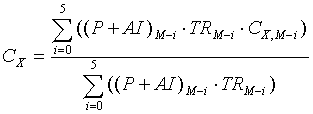WEIGHTED AVERAGE MATURITY DATE

The calculation of the weighted average maturity date (WAMD) of an MBS pool proceeds as follows. First, we calculate the weighted average number of days between the maturity date of the first tranche to mature, and the maturities of the remaining tranches. For a six tranche security, the calculation is as follows: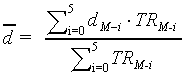where

M-i=maturity date of the tranche maturing i months before M
dM-i=number of days between the maturity date of the first tranche and M-i
TRM-i=remaining principal amount of tranche M-i

The weighted average maturity date is calculated by rounding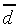to the nearest integer, then adding this weighted average number of days to the first tranche date:

WAMD=Maturity Date of First Tranche +Date Published: March 31, 2018